Open in App
Not now

# Volumes – Surface Area & Volumes | Class 9 Maths

• Difficulty Level : Hard
• Last Updated : 12 May, 2021

If an object is solid then space occupied by such an object is measured and termed as the Volume of the object. In short, the Volume of an object is the measure of the space it occupies, and the capacity of an object is the volume of substance its interior can accommodate. So, if we were to talk of the volume of a cube, we would be considering the measure of the space occupied by the cube.

The Unit of measurement of Volume is the cubic unit.

## Volume of Cube

A cube is a region of space formed by six identical square faces joined along their edges. Three edges join at each corner to form a vertex.

### Volume of Cube = edge x edge x edge = (a3) cubic units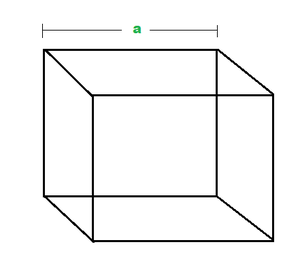Example 1: Find the volume of the cube, whose edge length is 3 cm?

Solution:

Volume of Cube = a3 (where a = edge or side length)
Volume = 3 x 3 x 3 = 27 cm

Example 2: Find the length of the side of the cube whose volume is 1331 cm3?

Solution:

Volume = side x side x side

=> 1331 = a3
Hence, side a = 11 cm.

## Volume of Cuboid

A cuboid is a box-shaped object. It has six flat faces and all angles are right angles. And all of its faces are rectangles.

### Where, l = length, w = width, h = height of Cuboid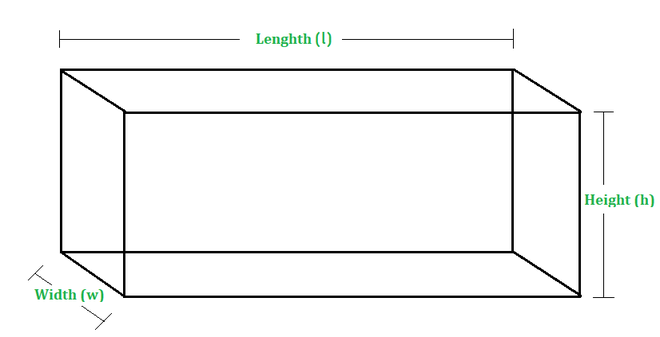Example 1: Find the volume of the cuboid whose length, width, and height are 10cm,11cm, and 13cm respectively?

Solution:

Volume of Cuboid = l x w x h = 10 x 11 x 13 = 1430 cm

Example 2: Calculate the length of the cuboid whose volume is given as 270-meter cube. And width and height are 6 and 9 meters respectively?

Solution:

Volume of cuboid = l x w x h

=> 270 = l x 6 x 9

=> l = 5 m

## Volume of Cylinder

Solids like measuring jars, circular pillars, circular pencils, circular pipes, road rollers, etc., are said to have a cylindrical shape.

### Where, r = base radius, h = height of cylinder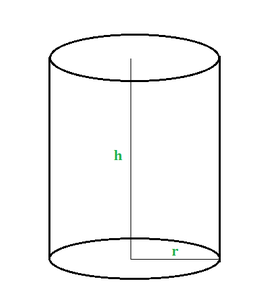Example 1: Find the volume of a cylinder whose radius is 10cm and height is 15cm. Take π = 22/7?

Solution:

Volume of Cylinder = πr2h = 22/7(10 x 10 x 15)

= 4714.28 cm3

Example 2: Find the height of a cylinder whose radius is 7cm and volume is 1540cm3.Take π = 22/7?

Solution:

Volume of Cylinder = πr2h

=> 1540 = 22/7(7 x 7 x h)

=> h = 10cm

## Volume of Cone

Solids like ice-cream cones, conical tents, funnels, etc., are having the shape of a cone.

### Where, r = radius of cone and, h = height of the cone.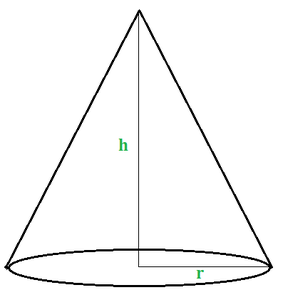Example 1: Find the volume of a cone whose radius is 7cm and height is 12cm. (π = 3.14)?
Solution:

Volume of Cone = 1/3(πr2h)

= 1/3(3.14 x 7 x 7 x 12)

= 615.44 cm3

Example 2: Determine the height of the cone whose volume and radius 308cm3, 7cm respectively. (π = 22/7)?
Solution:

Volume of cone = 1/3(πr2h)
=> 308 = 1/3(22/7 x 7 x 7 x h)
=> h = 6cm

## Volume of Sphere

Objects like a football, a cricket ball, etc., are said to have the shape of a sphere.

### Where, r is the radius of the sphere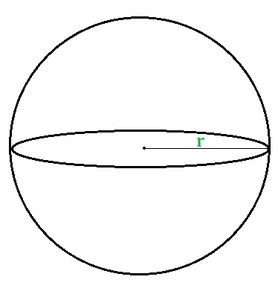Example 1: Find the volume of a sphere whose radius is 14cm. (π = 22/7)?

Solution:

Volume of Sphere = 4/3(πr3)
= 4/3(22/7 x 14 x 14 x 14)
= 11498.66 cm3

Example 2: Determine the radius of the sphere whose volume is 38808 m3?

Solution :

The volume of Sphere = 4/3(πr3)

=> 38808 = 4/3(22/7 x r3)
=> r = 21m

## Volume of Hemisphere

A plane through the centre of a sphere cuts it into two equal parts. Each part is called a hemisphere.

### Where, r is the radius of the hemisphere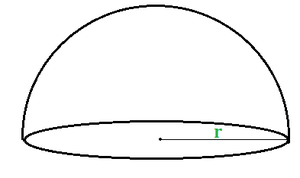Example 1: Find the volume of a hemisphere whose radius is 14 cm. (π = 22/7)?
Solution:

Volume of hemisphere = 2/3(πr3)
= 2/3(22/7 x 14 x 14 x 14)
= 5749.34 cm3

Example 2: Find the volume of a hemisphere whose radius is 7 cm. (π = 22/7)?

Solution:

Volume of hemisphere = 2/3(πr3)
= 2/3(22/7 x 7 x 7 x 7)
= 718.66 cm3

My Personal Notes arrow_drop_up